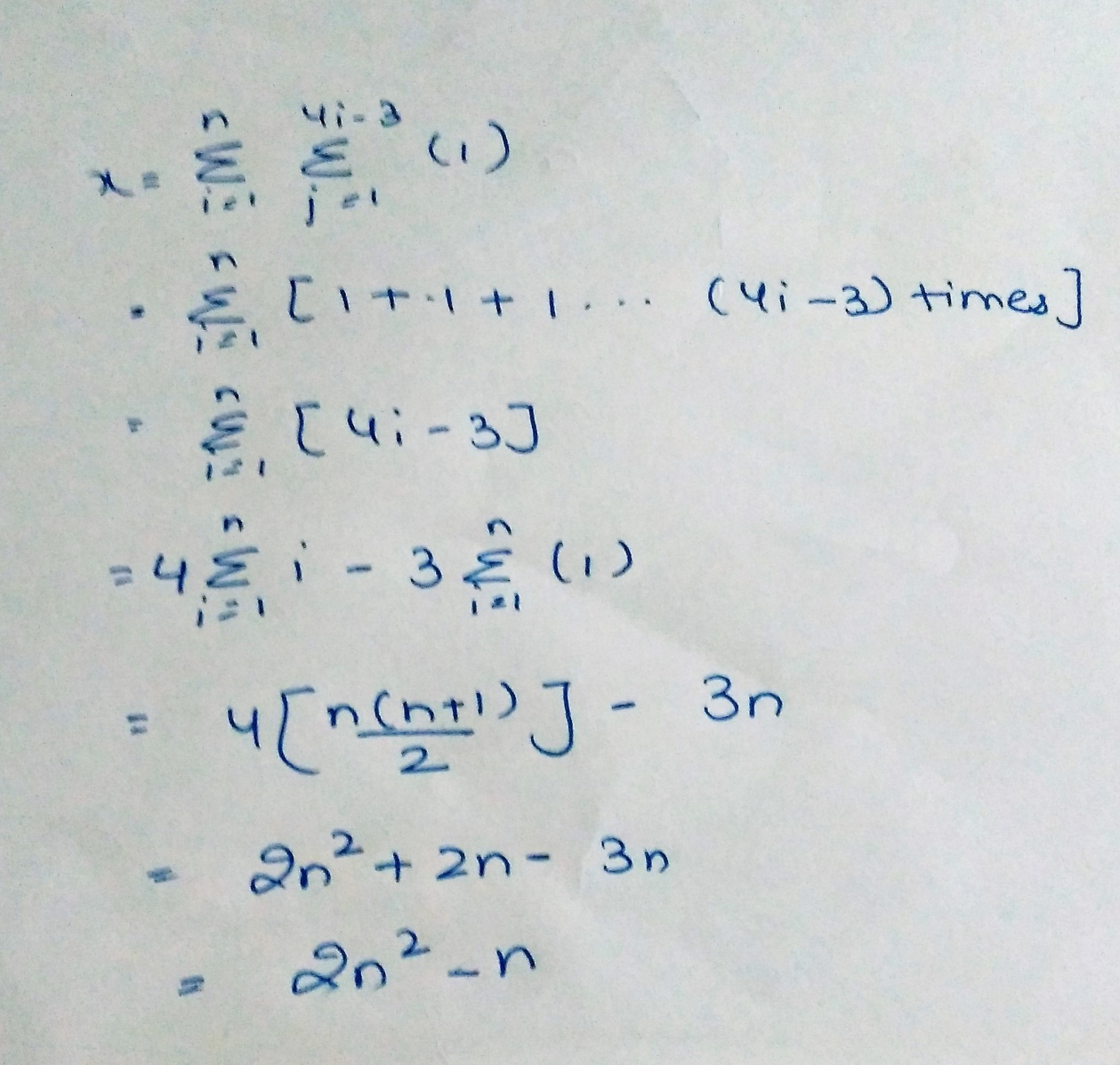Related Articles

# GATE | GATE 2017 MOCK II | Question 19

• Last Updated : 28 Jun, 2021

Consider the following pseudo code.

```x = 0;
for (i = 1 to n)
for (j = 1 to 4i – 3)
x = x + 1
```

Find the value of x, when the above code is executed in a function.

(A) n
(B) 2n2 – n
(C) 2n2 – 1
(D) 2n

Explanation: